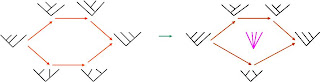## M Theory Lesson 99

Given an element $D$ of the descent algebra of $S_n$, let $Z(D)$ be the maximal permutation whose signature is $D$. Considering the natural order on the cube and permutohedra vertex sets, they become poset categories and $Z: Q_n \rightarrow P_n$ is a functor. In this paper it is shown that $Z$ has both a left and right adjoint. Moreover, the other pieces of the triangle

$Q_n \rightarrow A_n \rightarrow P_n$

also have left and right adjoints, giving a triple (cyclic) double adjunction involving these polytopes. This amazing categorical relation is a generalisation of an ordinary adjunction between just two categories. Since the associahedra were given as a 1-operad it is natural to try to view this triple as a 3 dimensional structure. Can we extend the use of vertices and edges to faces in a 2-category replacement for posets?Aside: Regarding the Hopf algebras here, P. Cartier provides the clearest exposition. MZVs appear on page 65.